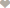Nigeria Student Forum

Welcome, Guest: Join Nigeria Student Forum / Login / Trending Now / Recent

Stats: 17,521 members, 69,862 Topics, 15,483 Comments. Date: Jun 27 2019, 9:00 am

Osorhor Ogenevwegba MichaelDelta state polytechnic otefe oghara ( DESPO )
Very interesting & meaningful msg 2 share:

If:
A B C D E F G H I J K LM N O P Q R S T U V W X Y Z
is equal to:

1 2 3 4 5 6 7 8 9 10 11 12 13 14 15 16 17 18 19 20 21 22 23 24 25 26

Do you agree that we have 26 alphabets in English, as given below

A = 1 ; B = 2 ; C = 3 ; D = 4 ;
E = 5 ; F = 6 ; G = 7 ; H = 8 ;
I = 9 ; J = 10 ; K = 11 ; L = 12 ;
M = 13 ; N = 14 ; O = 15 ; P = 16 ;
Q = 17 ; R = 18 ; S = 19 ; T = 20 ;
U = 21 ; V = 22 ; W = 23 ; X =24 ;
Y = 25 ; Z = 26.

Then,

H+A+R+D+W+O+R+K
=8+1+18+4+23+15+18+11
= 98%

K+N+O+W+L+E+D+G+E
=11+14+15+23+12+5+4+7+5
=96%

L+O+V+E
= 12+15+22+5
= 54%

L+U+C+K ;
=12+21+3+11
= 47%

None of them makes 100%. Then what makes 100%?

Is it Money?
.
NO!

M+O+N+E+Y
= 13+15+14+5+25
=72%

NO!

L+E+A+D+E+R+S+H+I+P
=12+5+1+4+5+18+19+8+9+16
=97%

Every problem has a solution, only if we perhaps change our.....

"ATTITUDE"

A+T+T+I+T+U+D+E ;
1+20+20+9+20+21+4+5
= 100%

It is therefore OUR ATTITUDE towards Life and Work that makes OUR Life 100% Successful.

Amazing Mathematics

Beautiful msg to share0 Like

Full Name:
Email: